### Image Composition Using Exposure Fusion

Brief Description

Files: \examples\Processing\HDR_ImageComposition\ExposureFusion\ExposureFusion.vaDefault Platform: mE5-MA-VCL

Short Description

An image composition from up to 16 images with different exposure times to one image showing details and contrasts over complete image without over- and under exposed pixels. This example is a simple alternative to High Dynamic Range Imaging.

Exposure fusion combines images with different exposure times to one image. In this resulting image under- and over exposed pixels are prevented and details are fully conserved. The aim of exposure fusion is similar to High Dynamic Range Imaging (see section and ), but in contrast to this method, the dynamic range of luminosity of the acquired images is not extended. Exposure fusion simply takes the best parts of the images of the sequence and combines them in one result image. Exposure fusion is easier to handle for the user, as he does not need to know the exposure times of the images acquired. The quality of the resulting image is slightly worse than with the algorithms of HDRI. In the following the exposure fusion algorithm, which is implemented in "ExposureFusion.va" is introduced.

#### Theory of Exposure Fusion

The exposure fusion algorithm according to [Mer07] combines images of a sequence with length N with a weighted blending to a result image :

Equation 44.

Here is the weighting for each color component red, green and blue of a pixel at position in the k-th image of the sequence with

Equation 45.

is a weighting function, which is dependent on the pixel value at position and is a measure for the well-exposedness of this pixel. In "ExposureFusion.va" is a linear function up to the pixel value of 127. For pixel values from 128 to 255, is set to 127. In dependence on his requirements the user easily can change this weighting function, as described in section e.g. according to [Mer07]. Quality measures like contrast or saturation (see [Mer07]) are not implemented in this reference design. In eq. 45 the sum over the color components red, green and blue in the denominator prevents, that a pixel in the result image looses the color information of the original images.

#### Implementation in VisualApplets

In Fig. 341 the basic design structure of "ExposureFusion.va" is shown.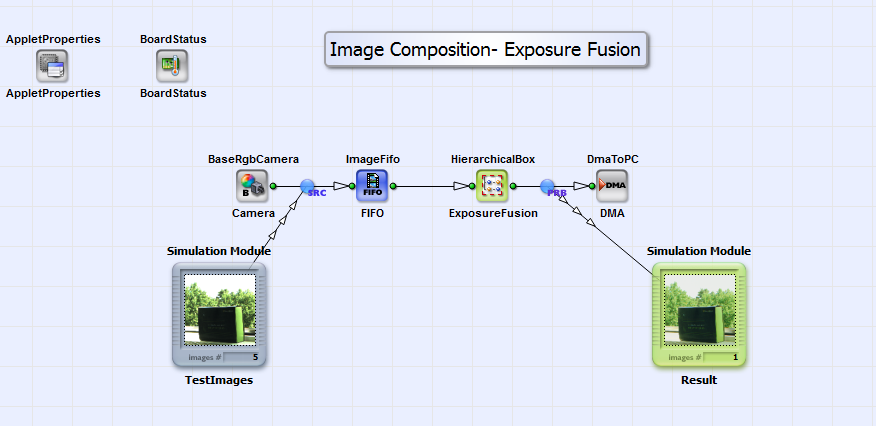Figure 341. Basic design structure of "ExposureFusion.va"

The images of a sequence from an rgb camera in Camera link base configuration are combined to one result image according to the algorithm in eq. 44 in the HierachicalBox ExposureFusion. For simulation purpose please load up to 16 images of maximum dimensions of 1024x1024 pixels to the simulation source TestImages. The order of the images with respect to their exposure times is not of importance! With "right-mouse-click" on box ExposureFusion you can set the image sequence length and the image dimensions of the input images. The maximum number of images is 16 and the maximum image dimensions are 1024x1024 pixels. The output image is sent via DMA to PC. In Fig. 342 the content of the HierarchicalBox ExposureFusion is shown.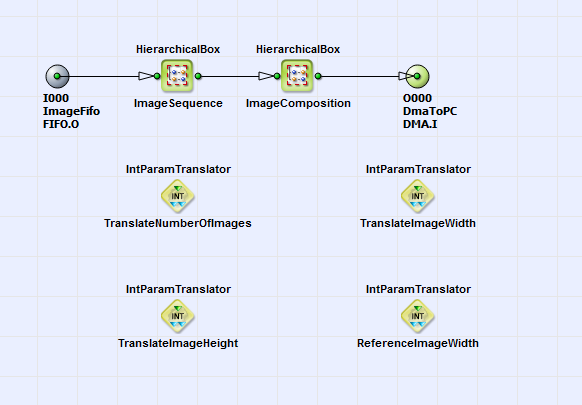Figure 342. Content of HierarchicalBox ExposureFusion

In the box ImageSequence the images from the sequence are combined to one large image. The pixels at position from the images are positioned as neighbors. In the box ImageComposition the exposure fusion algorithm according to eq. 44 is implemented. You can see its content in Fig. 343.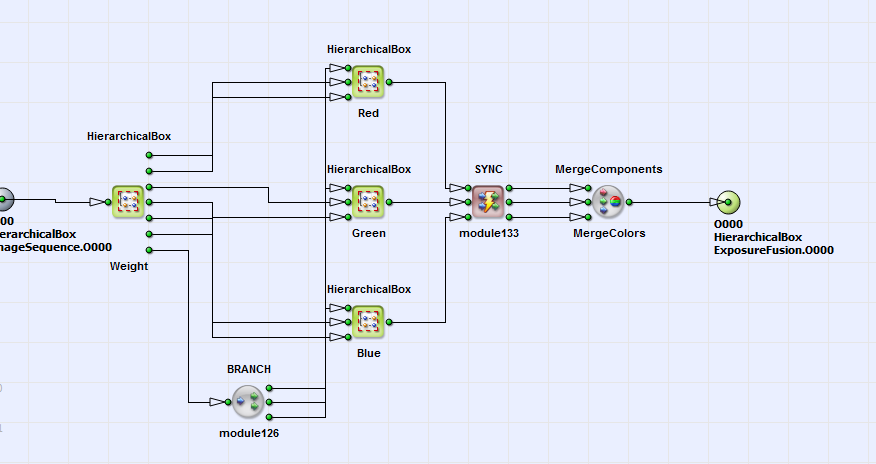Figure 343. Content of HierarchicalBox ImageComposition

In box Weight the weighting function (see. eq. 45) in dependence of the pixel value is implemented for each color component. You can see the content of box Weight in Fig. 344.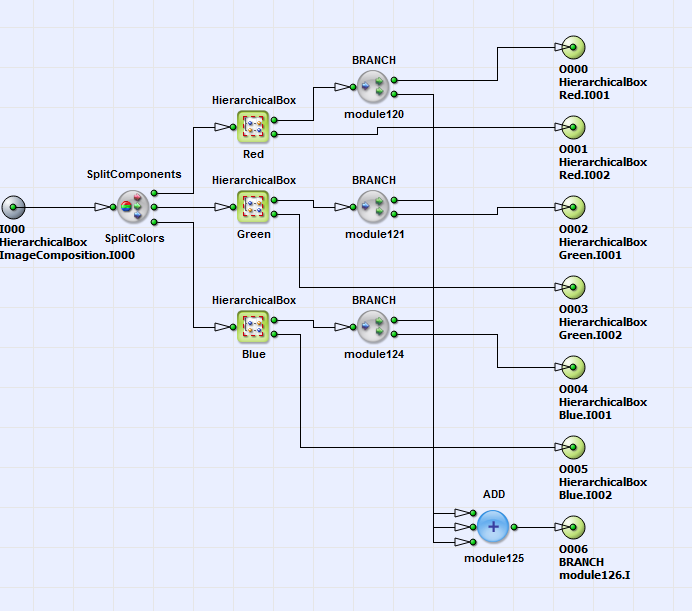Figure 344. Content of HierarchicalBox Weight

The weighting functions for each color component from boxes Red, Green and Blue are summed up, according to eq. 45. In Fig. 345 you can see the weighting function for the color red.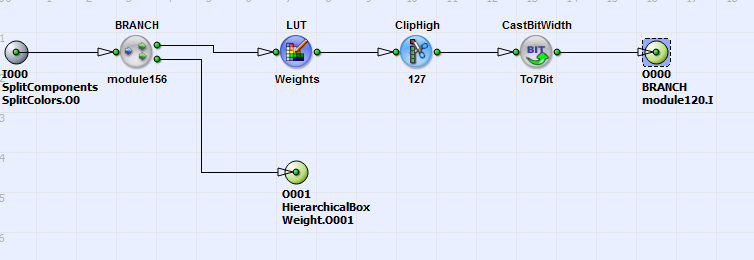Figure 345. Content of HierarchicalBox Red in box Weight

Up to a pixel value of 127 the function is linear. From pixels values 128 to 255, is set to 127. The lookup table operator LUT_Weights allows the user to adapt the weighting function easily to his requirements. Also changing the parameters for the ClipHigh and CastBitWidth operators (or even deleting them) gives the user this possibility. Coming back to the content of box ImageComposition. Here according to eq.44 the pixel values for the color components red, green and blue are multiplied with the weighting function and with a constant Const of value 3 in the boxes Red, Green and Blue. You can see the content of box Red in Fig. 346.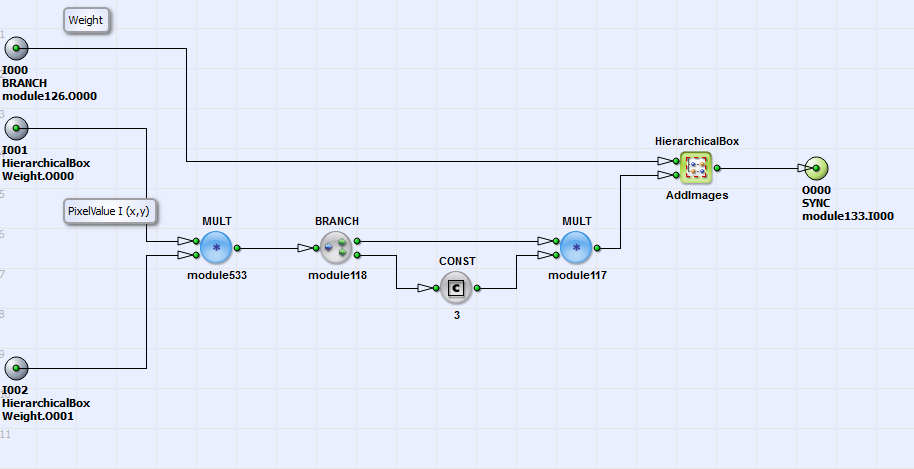Figure 346. Content of HierarchicalBox Red in box ImageComposition

Here is summed up for all images. is then divided by according to eq. 45. Finally the color components are merged together (see Fig. 343) and the output image is sent to PC. In Fig. 347 you can see 5 example input images with the result image in Fig. 348. The result image has no under- and over exposed pixels and details are conserved.Figure 347. Example input images with different exposure times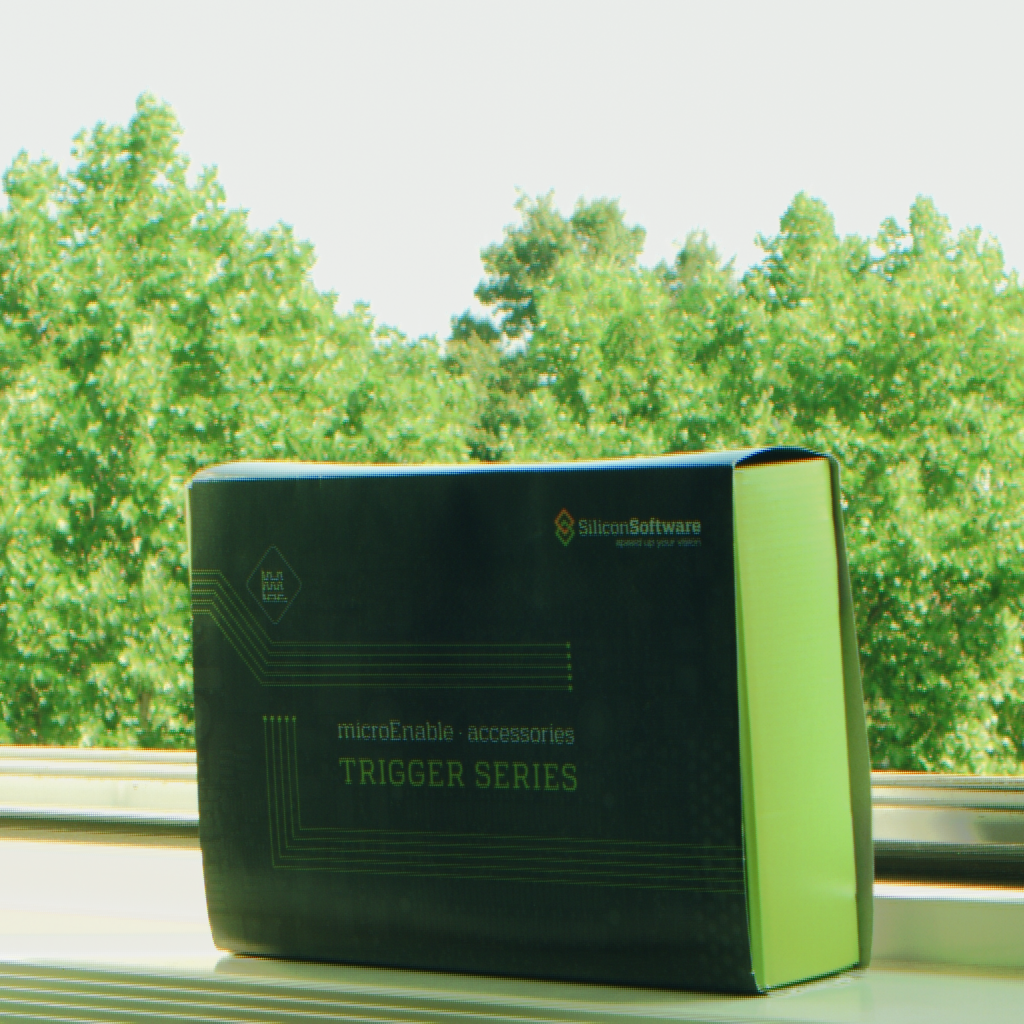Figure 348. Result image of the 5 example input images after exposure fusion